# Mathematical Modelling and Analysis of Hybrid Solar Desalination System Using Evacuated Tube Collector (ETC) and Compound Parabolic Concentrator (CPC)

Mathematical Modelling and Analysis of Hybrid Solar Desalination System Using Evacuated Tube Collector (ETC) and Compound Parabolic Concentrator (CPC)
Department of Mechanical Engineering, Koneru Lakshmaiah Education Foundation, Green Field Vaddeswaram, Guntur 522502, India

Department of Mechanical Engineering, MKSSS’s Cummins College of Engineering for Women, Pune 411028, India

Corresponding Author Email:
shridhar.kedar@cumminscollege.in
Page:
45-51
|
DOI:
https://doi.org/10.18280/mmep.080105
17 September 2018
|
Accepted:
6 November 2020
|
Published:
28 February 2021
| Citation

OPEN ACCESS

Abstract:

Solar desalination system is one of the technologies which converts hard water to soft water which even is useful for drinking purpose. This paper reports the mathematical modelling of hard water desalination using hybrid (ETC + CPC) solar desalination system. This model considers various parameters such as intensity of solar radiation, atmospheric temperature, wind speed, material for compound parabolic concentrator, flow rate, concentration ratio and gives idea for system performance in terms of soft water production. Experiments were conducted at Pune City which is located in western region of India and accordingly environmental parameter are considered accordingly.  It is observed that average percentage error values calculated using this proposed model to quantity soft water in comparison with actual experimental results are in the range of 3% to 5%. Hence, it is concluded that numerically calculated quantity of soft water per bottle has good agreement with analytical calculation with developed thermal model. It is evident that developed model predicts the behaviour of the actual system.

Keywords:

evacuated tube solar collector, compound parabolic concentrator, condenser, solar desalination system

1. Introduction

Now a days evacuated tube solar collector and compound parabolic concentrator are widely used for heating of fluids with the help of Solar Energy. Evacuated glass tube is made up of borosilicate glass with outer surface of inner tube coated with selective coating (Al-Ni) for the maximum amount of solar energy absorption and heat loss minimum. Through the solar rays enters to outer evacuated glass tube, heat is generated inside the inner tube with help of selective black coating (Al-Ni). Compound parabolic concentrator made up of aluminium sheet to increase the overall heating performance of solar desalination system. Earlier researcher had developed different mathematical model based on mass and energy conservation. This paper reports as an attempt to developed hybrid mathematical model for desalination system.

Mathematical model of solar desalination system investigated by Many researchers. Sapre et al.  worked on design and manufacturing of absorber for solar desalination system. Kumar et al.  studies on thermal performance one end evacuated tube for producing hot air. An experimental model was prepared for generation of hot air. Al-Tabbakh et al.  reported that experimental analysis of evacuated tube solar water collector with surpentive flow through pipe. Their study shows that to find out applicability to produce high temperature water or steam whereas Hunashikatti et al.  develops desalination unit using solar still coupled with evacuated tubes for domestic application.The chemical analysis of water samples were done as per IS 10500:2012 (Drinking water specification). Dhamsa-ard et al.  they found that monthly average energy of the compound parabolic concentrator produced through out the year is equal to 286.16 kWh or equal to 3433.87 kwh/year whereas Yadav and Bajpai  experimentally investigated thermal performance of one end evacuated tube solar air collector at different flow rates. Mayur patel and krunal patel  mainly studies critical review of evacuated tube collector whereas Dabra et al.  were experimentally investigated effect of tilt angle on the performance of evacuated tube solar air collector. Tilt angle indicated that no positive effect on the thermosyphon phenomenon inside the evacuated tubes whereas Kedar et al.  mainly studies thermal analysis of an evacuated tube collector in solar desalination system. Author mainly focus on single effect boiling method for ground water solar desalination system. Reddy et al.  had done performance analysis of evacuated multi stage solar water desalination system. They argued that multi stage evacuated solar desalination system is a viable option to meet the need of rural and urban communities whereas Siddiqui et al.  studies experimentally and theoretically performance improvement solar desalination system by using solar air heater. Kedar et al.  experimentally investigated solar desalination system using evacuated tube collector whereas

Solar water heating using heat pipe with ETC was studied by Sakthivel et al.  and analytical model was proposed.

Borah et al.  developed compound parabolic concentrator to increase the solar intensity for effective temperature, they were argued that solar radiation trapping time at constant temperature level was increased by 1.5 hours in comparision with to fixed compound parabolic concentrator whereas Murali et al.  focused on experimental study of thermal performance of solar aluminium cane air heater with and without fins Further, Kedar et al. focused on design and analysis of use of CPC for ground water desalination application was recommended [16, 17].Whereas Murali et al.  on the basis of experimental studies optamized the design of solar aluminium air heater with CPC for with and without latent heat storage conditions. Murali et al. performed thermal analysis of One Slope Solar Unit Conjoin with Parabolic Concentrator [18, 19]. Saettone  had investigated solar desalination using Parabolic Through Concentrator, this study shows a good quality of fresh water obtained using parabolic through concentrator.

Moh’d et al.  developed hybrid solar wind water desalination system, they argued that the yield can be three to four times more than conventional solar distillers. Their system mainly base on single basin solar still and a wind water heater whereas Kedar et al. developed on performance analysis of hybrid solar desalination system using ETC and CPC [22-24], whereas Kabeel et al.  were studies on review for water desalination using humidification and dehumidification techniques. This review would also be light on the scope further research and recommendations on active distillation system whereas Kalogirou  studies that seawater desalination using renewable energy sources. This paper mainly includes review of various system using renewable energy sources for desalination.

It is observed from literature survey that, most of the studies carried out by earlier researcher for the different analytical, experimental and numerical investigation. Their primary focus was to predict thermal performance of evacuated tube, compound parabolic concentrator,parabolic trough collector, absorber, wind turbine for solar desalination system. The numerical studies related to solar and wind hybrid system for desalination were reported into literature. However due to inherent limitations of experimental measurements and maximum availability of drinkable soft water through (ETC+CPC) hybrid solar ground water desalination system is not found in this literature. Along with that the study was carried out for different climatic and environmental conditions of Pune city which is located Weston region in Maharashtra. The important aim of this mathematical model in hybrid (ETC+CPC) is that to develop an equation to quantify quantity of soft water produced using the said hybrid solar ground water desalination system. This mathematical model significantly analyses the effect of different parameters on heat transfer. Section II discuss Mathematical modeling of hybrid solar desalination system.

The paper is organised in five sections. Section one has presented relevance and review. Section two discuss mathematical modelling of hybrid solar desalination system. Section three discuss numerical solution with MATLAB program. Section four discuss results and discussions and conclusions are reported in section number five.

2. Mathematical Modeling of Hybrid Solar Desalination System

Thermal model is developed for solar desalination system under atmospheric conditions of Pune city. For solar desalination system hard water is used working fluid which is easily available in to the nature. In case of hard water hardness in the rage of 270 to 400 mg/litre. Through the entire developed system all possible parameters will be control with specified limits as per IS standard 10500:2012 drinking water specification. The developed mathematical model depends upon on the energy conservation. In particular mathematical modelling serves the purpose the for collector runs significantly. This paper reports is an attempt to develop mathematical model which mainly consist of heat absorbed by heat absorbed by ETC and CPC, total exposed area of collector, heat loss to surrounding by solar collector and net heat gain by solar collector.

Following assumptions are made for Mathematical model for hybrid energy system

1) Evacuated tube collector and compound parabolic concentrator is undergoing to steady state.

2) Solar diffused radiation on the actual system is neglected.

3) Heat flow through back insulation is less.

4) Heat flow through ETC glass tube and CPC will be one dimensional.

5) There is no shading on ETC and CPC.

6) There is no heat loss for the entire system.

Based on these assumptions mathematical model developed in hybrid solar desalination system.

The flow of evacuated tube collector is completely closed in addition to that copper tubes inserted inside the tubes. The main purpose of the copper tubes, copper has high thermal conductivity 380 w/mK, with latent heat of evaporation 4796 J/g and latent heat of fusion 205 J/g and maximum heat storing capacity. On one side hard water comes into the tubes and another side steam passing through to the condenser. Their use as a first wall material has been proposed in designs where high thermal loads are expected on the first wall or where a shell of high electrical-conductivity material is required. Copper alloys have also been considered for the electrically-conducting material. The following equations are considered for mathematical modelling in evacuated tube collector and compound parabolic concentrator. Figure 1 shows thermal model of evacuated tube collector with Cu tubes whereas Figure 2 shows a CAD model of compound parabolic concentrator.

The quantity of heat absorbed by Evacuated tube collector

$Q a b s=A c \times I G \times \eta o p t(J / s e c)$     (1)

Total area exposed to collector

$A c=\Pi \times D \times L \times N$     (2)

Heat loss to surrounding by solar collector

$Qloss =QCond +QConv +Qref (\mathrm{J} / \mathrm{sec} .)$     (3)

Net Heat gain by collector

$Q u=Q a b s-Q \operatorname{loss}(J / s e c)$     (4)

Hybrid mathematical modelling equations

$Heat absorbed by ETC and \mathrm{CPC}=(\mathrm{Qabs}+\mathrm{Qu})$$=(A c \times I g \times \eta o p t)+(A c * \operatorname{Fr}(S-U L(T i-T a)))$$=A c(I g \times \eta o p t)+(F r(S-U L(T i-T a)))$     (5)

Total area exposed to collector ETC and CPC$=\Pi \times D \times L \times N(m 2)$

Heat loss due to surrounding solar collector$=($ Qloss $)=($ Qcond $+$ Qconv $+$ Qref $)$     (6)

## 1.png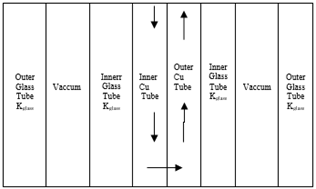Figure 1. Thermal model of evacuated tube collector with Cu Tubes

## 2.png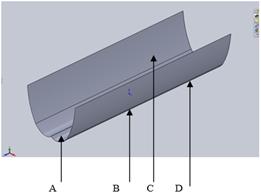A: Absorber area, B: Concentration line of CPC, C: Compound parabola, D: Compound parabolic concentrator

Figure 2. Compound parabolic concentrator model

Neat heat gain by the collector = Qu = Heat absorbed by collector – Heat loss by collector.

Considered the flow in evacuated tube collector and compound parabolic concentrator is completely.

Enclosed, energy balance equation can be applied to determine the variation in mean temperature of working fluid. Hence heat gain by the working fluid equation,

Heat gain by the working fluid $=c f\left(\frac{\delta}{\delta t T f}+\frac{u \delta}{\delta x T f}\right)$$+C R(\delta / \delta x T f+u \delta / \delta x T f) (7) The outer glass tube temperature is calculated as: C g\left(\frac{\delta T g}{\delta t}\right)=\varepsilon g \sigma(T a 4-T g 4)$$+\varepsilon g \sigma(\operatorname{Tr} 4-T f 4)+H c o n g-a(T g-T a)$     (8)

This equation stats the change in cover temperature which is proportional to the difference between net heat gain rate by the receiver to the heat losses to the environment by radiation and convection.

The convective heat transfer coefficient between the atmosphere and the outer glass is given by the equation, $H_{\text {con }}^{g-a}=5.678+3.8 v$.

Compound Parabolic concentrator is a radiation concentrator of the fixed concentrator having moderate temperature in the range of 100-300℃ with solar radiation reflector. The main purpose for using compound parabolic concentrator to utilize solar heat and reverse back to evacuated tube collector bottom side for maximum amount of soft water formation within less time. The above equations are used in compound parabolic concentrator.

Concentration ratio depending on the medium between reflector area and acceptance half angle to be given by equation, CR2- dimension $=(n / \sin \theta c)$ total height of Compound parabolic concentrator (H), focal length (f), acceptance half angle (θc), Circumference of the absorber (a).

$H=a / 2((1 / 2)+(1 / \pi \sin \theta c)+((1) /(\sin \theta \cdot \tan \theta c))$

Equation of the parabolic arc,

$B C=(d / 2)[(\beta+\theta c+\pi / 2)-\cos (\beta-\theta c)] /(1+\sin ((\beta-\theta c))$

Using the above equations, selected parameters such as intensity of solar radiation, mass flow rate, absorber area, density and specific heat of fluid and temperature are considered for modelling, Using Buckingham’s π theorem π1, π2, π3 equations are to be generated with the help of regression analysis analysis to form the equation in the form of mass flow rate of water for solar desalination.

Quantity of soft water will be mainly depending on intensity of solar radiation, flow rate of water, heat transfer rate in water to steam and steam to water.

$m=1.802 \times 10^{-6} \times(\rho \times k) \times\left(Q^{0.605} \times I^{0.21} \times d^{0.22}\right) \times(y \times \theta)^{0.185} /\left(\sum A a b s^{0.2025}\right)$     (9)

Hence rewrite this equation in the form of Q i.e quantity of soft water for desalination system

$Q=m \times\left(\sum A a b s^{0.2025}\right) /\left\{1.802 * 10^{-6} \times(\rho k) \times\left(I^{0.21} \times d^{0.22}\right) \times(y \times \theta)^{0.185}\right\}^{(1 / 0.605)}$     (10)

In our research the main output parameter was Q i.e quantity of soft water which is mainly depends upon intensity of solar radiation, density of fluid, quantity of heat absorbed, absorber area of the evacuated tube and compound parabolic concentrator.

Finite difference method is used to solve this system. MATLAB program is developed to solve this set of equations. Through MATLAB programming predicting the results, at the first iteration boundary condition like the glass temperature Tg and ambient temperature Ta is considered as a same.

3. Numerical Solution with Matlab Program

MATLAB program is developed to calculate geometrical parameters and performance characteristics of CPC which is to be designed at any given location. The input required for this program is geographical location parameters, ETC geometrical specifications, solar constants required for predicting hourly global radiation etc. The output of the program gives the geometrical parameters of CPC and the heat analysis of CPC. The flowchart (Figure 3) of the program implemented in MATLAB to calculate the quantity of soft water for desalination system.

A validated model with the help of MATLAB program it is possible to analyse the effect of different climatic conditions of western region of India and various performance of the system by using thermal model under steady state condition.

## 3.png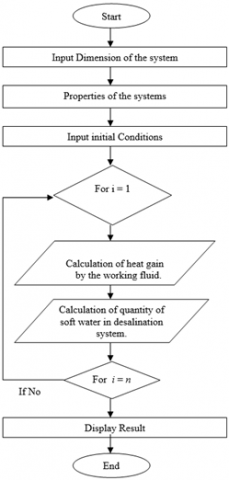Figure 3. Flowchart of MATLAB program to calculate the quantity of soft water for desalination system

4. Results and Discussions

The hybrid mathematical modelling we can predict the quantity of soft water for the desalination system. It mainly depends upon mass flow rate of water, absorber area of collector, collector outlet temperature etc. The following equation helps us for calculating quantity of soft water in desalination system.

$Q=m \times\left(\Sigma A a b s^{0.2025}\right) /\left\{1.802 * 10^{-6} \times(\rho k) \times\left(I^{0.21} \times d^{0.22}\right) \times(y \times \theta)^{0.185}\right\}^{(1 / 0.605)}$

Figure 4 shows the graph of temperature Vs time for a day 1st May 2018.The graph shows maximum collector outlet temperature as 82℃. Figure 5 shows soft water in liters Vs time (entire day), it shows system could produce 1.7 liters soft water/day.

Figure 6 shows the graph of temperature Vs time for a day 2nd May 2018.This graph shows maximum collector outlet temperature 84℃. Figure 7 shows soft water in liters Vs time (entire day), it shows system could produce 1.8 liters soft water/day.

Figure 8 shows that graph of temperature Vs time for a day 14th May 2018. The graph will show maximum collector outlet temperature 83℃. Figure 9 shows soft water in liters Vs time (entire day), it shows system could produce 1.75 liters soft water/day.

Figure 10 shows that graph of intensity of solar radiation Vs time. The graph will show maximum intensity of solar radiation 930 W/m2 at 13:00 hours.

Figure 11 shows the comparative analysis of analytical prediction and experimentally collected soft water during a period of one month. Figure 12 shows the comparative analysis of analytical predection and exprimentally obtained collector outlet average temperature water during a period of one month.

Figure 13 shows that ambient temperature through a month in Pune city. Average ambient temperature range observed 27-30℃. Figure 14 shows intensity of solar radiation through a month in Pune city.

## 4.png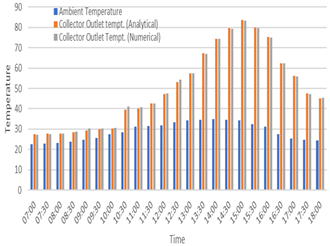Figure 4. Graph of Temperature Vs Time for a day (01/05/2018)

## 5.png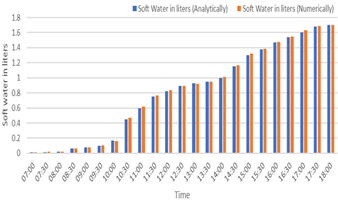Figure 5. Graph of Soft water in liters Vs Time for a day (01/05/2018)

## 7.png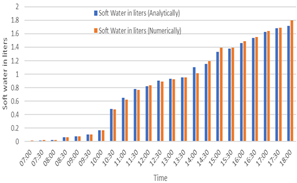Figure 6. Graph of Temperature Vs Time for a day (02/05/2018)

## 7.pngFigure 7. Graph of Soft water in liters Vs Time for a day (02 /05/2018)

## 8.png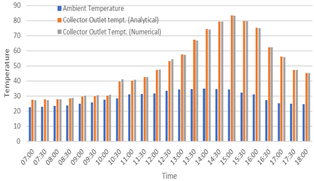Figure 8. Graph of Temperature Vs Time for a day (14 /05/2018)

## 9.png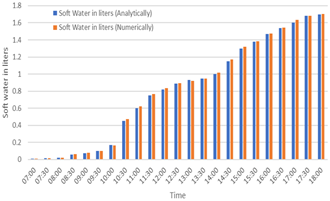Figure 9. Graph of Soft water in liters Vs Time for a day (14 /05/2018)

## 10.png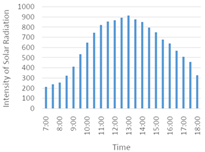Figure 10. Intensity of Solar radiation with time

## 11.png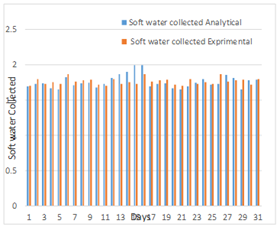Figure 11. Soft water collected analytically and exprimentally throughtout a month

## 12.png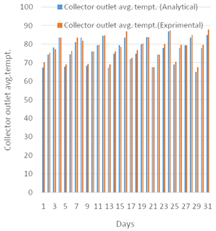Figure 12. Collector outlet average temperature analytically and exprimentally throught a month

## 13.png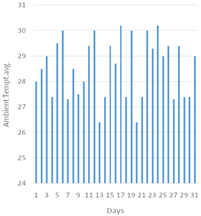Figure 13. Ambient temperature throught a Month in Pune city

## 14.png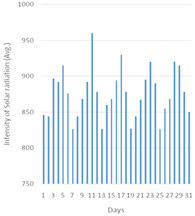Figure 14. Intensity of solar radiation throught a Month in Pune city

Through this mathematical model the analytical data to be calculated for each half interval time solar radiation, ambient temperature, collector outlet temperature and soft water in liters. This analytical analysis shows that the outlet temperature of water by lesser than the numerical value. As a result, the heat which is to be absorbed by water gets reduced. The graphs are plotted to show the time of evaporation required for different quantities of water and the average outlet temperature variation with respect to time. Also the graph time Vs solar radiation shows that morning 10:00 am to afternoon 15:00 pm get the good intensity of solar radiation to get the maximum quantity of soft water. Due to these results it is concluded that collector outlet working temperature and soft water collected has a good agreement with developed hybrid mathematical model.

5. Conclusion

This paper reports mathematical modelling and analysis of hybrid solar desalination system using evacuated tube collector and compound parabolic concentrator. Mathematical model of hybrid evacuated tube collector and compound parabolic concentrator is developed with considering relevant data pertaining to the Pune city which is located in western region of India. The thermal analysis shows combined effect of temperature in evacuated tube collector and compound parabolic concentrator observed in the range of 140-145℃ for the environmental and climatic conditions of Pune city. In case of this theoretical and experimental analysis by assuming flow rate of 2-7 LPH in single ETC and CPC system gives approximately 1.5 to 2.5 liters of soft water per day. For solving differential equations MATLAB programming is done and results are obtained. It is observed that average percentage error values calculated using developed model to quantity soft water in comparison with actual experimental results are in the range of 3% to 5%. Hence, it is concluded that numerically calculated quantity of soft water per bottle has good agreement with analytical calculation using developed thermal model. This analysis may form baseline of further research to improve the thermal performance of evacuated tube collector with copper tubes and compound parabolic concentrator by reducing time.

Acknowledgment

The project (15ENG000570) supported by Board of college and University Development (BCUD) Savitribai Phule Pune University (SPPU), Pune and Cummins College of Engineering for Women are gratefully acknowledged.

Nomenclature
 d Inner diameter of Tube D Outer diameter of Tube B Internal surface between tubes L Length of the Tube t Glass thickness A Absorber area τ Normal Projection factor a Normal absorption factor UL Heat loss coefficient FR Heat loss factor T Temperature V Wind Velocity I Intensity of solar radiation Hd Global solar radiation Ho Direct solar radiation Kt Clearness Index Ca Calcium F Fluorine Mg Magnesium TDS Total Dissolved Solids Qabs Heat absorbed in kJ Qcond Conduction heat loss in kJ Qconv Convention heat loss in kJ Qref Reflection heat loss in kJ Qloss Heat loss in kJ Qu Useful heat in kJ H Total height of CPC in mm Θ Air solar heater absorber groove angle Θc Acceptance half angle ETC Evacuated Tube Collector CPC Compound Parabolic Concentrator
References

 Sapre, M.S., Auti, A.B., Singh, T.P. (2014). Design and manufacturing of absorber for solar desalination system. Applied Mechanics and Materials, 446: 716-720. https://doi.org/10.4028/www.scientific.net/AMM.446-447.716

 Kumar, A., Kumar, S., Nagar, U., Yadav, A. (2013). Experimental study of thermal performance of one-ended evacuated tubes for producing hot air. Journal of Solar Energy. http://dx.doi.org/10.1155/2013/524715

 Al-Tabbakh, L.D.A.A., Mohammed, A.L.A.A. (2014). Experimental investigation of an evacuated-tube solar water collector with serpentine through-flow pipe. Journal of Engineering and Sustainable Development, 18(2): 33-40.

 Hunashikatti, P.T., Suresh, K.R., Prathima, B., Sachdeva, G. (2014). Development of desalination unit using solar still coupled with evacuated tubes for domestic use in rural areas. Current Science (00113891), 107(10): 1683-1693.

 Chamsa-ard, W., Sukchai, S., Sonsaree, S., Sirisamphanwong, C. (2014). Thermal performance testing of heat pipe evacuated tube with compound parabolic concentrating solar collector by ISO 9806-1. Energy Procedia, 56: 237-246. https://doi.org/10.1016/j.egypro.2014.07.154

 Yadav, A., Bajpai, V.K. (2012). Thermal performance of one-ended evacuated tube solar air collector at different flow rates: Experimental investigation. International Journal of Ambient Energy, 33(1): 35-50. https://doi.org/10.1080/01430750.2011.636207

 Patel, M., Patel, K. (2013). A critical review of Evacuated tube colletor. International Journal of Advanced Engineering Research and Studies, 2(3): 55-56.

 Dabra, V., Yadav, L., Yadav, A. (2013). The effect of tilt angle on the performance of evacuated tube solar air collector: experimental analysis. International Journal of Engineering, Science and Technology, 5(4): 100-110.

 Kedar, S.A., Kumaravel, A.R., Bewoor, A.K. (2019). Experimental investigation of solar desalination system using evacuated tube collector. International Journal of Heat and Technology, 37(2): 527-532. https://doi.org/10.18280/ijht.370220

 Reddy, K.S., Kumar, K.R., O'Donovan, T.S., Mallick, T.K. (2012). Performance analysis of an evacuated multi-stage solar water desalination system. Desalination, 288: 80-92. https://doi.org/10.1016/j.desal.2011.12.016

 Siddiqui, F.R., Elminshawy, N.A., Addas, M.F. (2016). Design and performance improvement of a solar desalination system by using solar air heater: Experimental and theoretical approach. Desalination, 399: 78-87. https://doi.org/10.1016/j.desal.2016.08.015

 Kedar, S.A., Raj, K.A., Bewoor, A.K. (2018). Thermal analysis of solar desalination system using evacuated tube collector. AIP Conference Proceedings, 2039(1): 020061. https://doi.org/10.1063/1.5079020

 Sakthivel, M., Reddy, P.R. (2019). Analytical investigations of solar water heating using heat pipe with evacuated tubes. International Journal of Innovative Technology and Exploring Engineering, 8(8): 1938-1945.

 Borah, A., Khayer, S.M., Sethi, L.N. (2013). Development of a compound parabolic solar concentrator to increase solar intensity and duration of effective temperature. International Journal of Agriculture and Food Science Technology, 4(3): 161-168.

 Murali, G., Sundari, A.T.M., Raviteja, S., Chanukyachakravarthi, S., Tejpraneeth, M. (2020). Experimental study of thermal performance of solar aluminium cane air heater with and without fins. Materials Today: Proceedings, 21: 223-230. https://doi.org/10.1016/j.matpr.2019.04.224

 Kedar, S.A., Raj, K.A., Bewoor, A.K. (2018). Design and analysis of solar desalination system using compound parabolic concentrator. IOP Conference Series: Materials Science and Engineering, 455(1): 012063. https://doi.org/10.1088/1757-899X/455/1/012063

 Kedar, S.A., Bewoor, A.K. (2019). Patent on “Solar Desalination System” Ref. No.201921032482. The Patent office Journal No 07/2021, PP 7767, Application Number, TEMP/E-1/34303/2019-MUM.

 Murali, G., Sandeep, G., Hari, N., Praveen, M., Neeraj, B. (2018). Experimental studies on solar aluminium can air heater with and without latent heat storage. International Journal of Mechanical Engineering and Technology, 9(4): 145-155.

 Swapna Babu, B., Murali, G. (2019). Performance study on one slope solar unit conjoin with parabolic concentrator. International Journal of Innovative Technology and Exploring Engineering, 8(8): 1452-1455.

 Saettone, E. (2012). Desalination using a parabolic-trough concentrator. Applied Solar Energy, 48(4): 254-259. https://doi.org/10.3103/S0003701X12040081

 Moh'd A, A.N., Kiwan, S.M., Talafha, S. (2016). Hybrid solar-wind water distillation system. Desalination, 395: 33-40. https://doi.org/10.1016/j.desal.2016.05.018

 Kedar, S.A., Raj, K.A., Bewoor, A.K. (2019). Performance analysis of hybrid solar desalination system using ETC and CPC. SN Applied Sciences, 1(9): 965. https://doi.org/10.1007/s42452-019-0985-3

 Kedar, S.A., Murali, G., Bewoor, A.K. (2021). Effective hybrid solar groundwater desalination in rural areas. International Transaction Journal of Engineering, Management & Applied Sciences & Technologies, 12(3): 1-10. https://doi.org/10.14456/ITJEMAST.2021.55

 Kedar, S.A., Bewoor, A.K., Madhusudan, S. (2016) Solar desalination system using evacuated tube collector & compound parabolic concentrator- theoretical approach. National Conference on Advancement in Electrical Engineering and Energy Sciences.

 Kabeel, A.E., Hamed, M.H., Omara, Z.M., Sharshir, S.W. (2013). Water desalination using a humidification-dehumidification technique-A detailed review. Natural Resources, 4(3): 286-305. https://doi.org/10.4236/nr.2013.43036

 Kalogirou, S.A. (2005). Seawater desalination using renewable energy sources. Progress in Energy and Combustion Science, 31(3): 242-281. https://doi.org/10.1016/j.pecs.2005.03.001